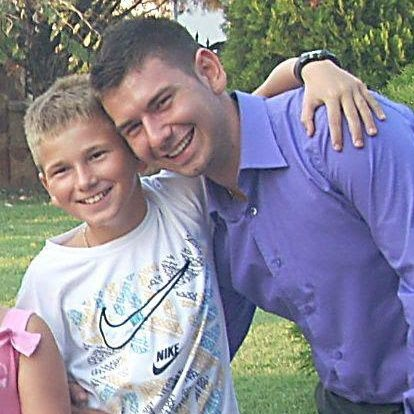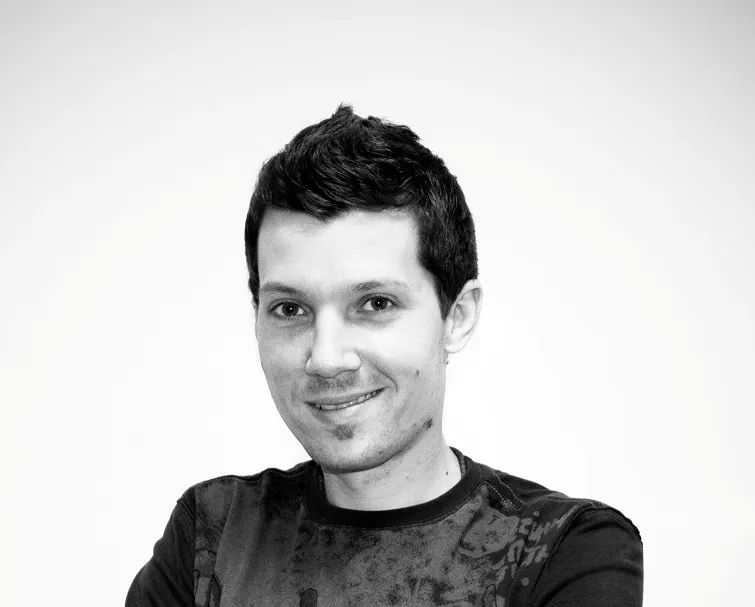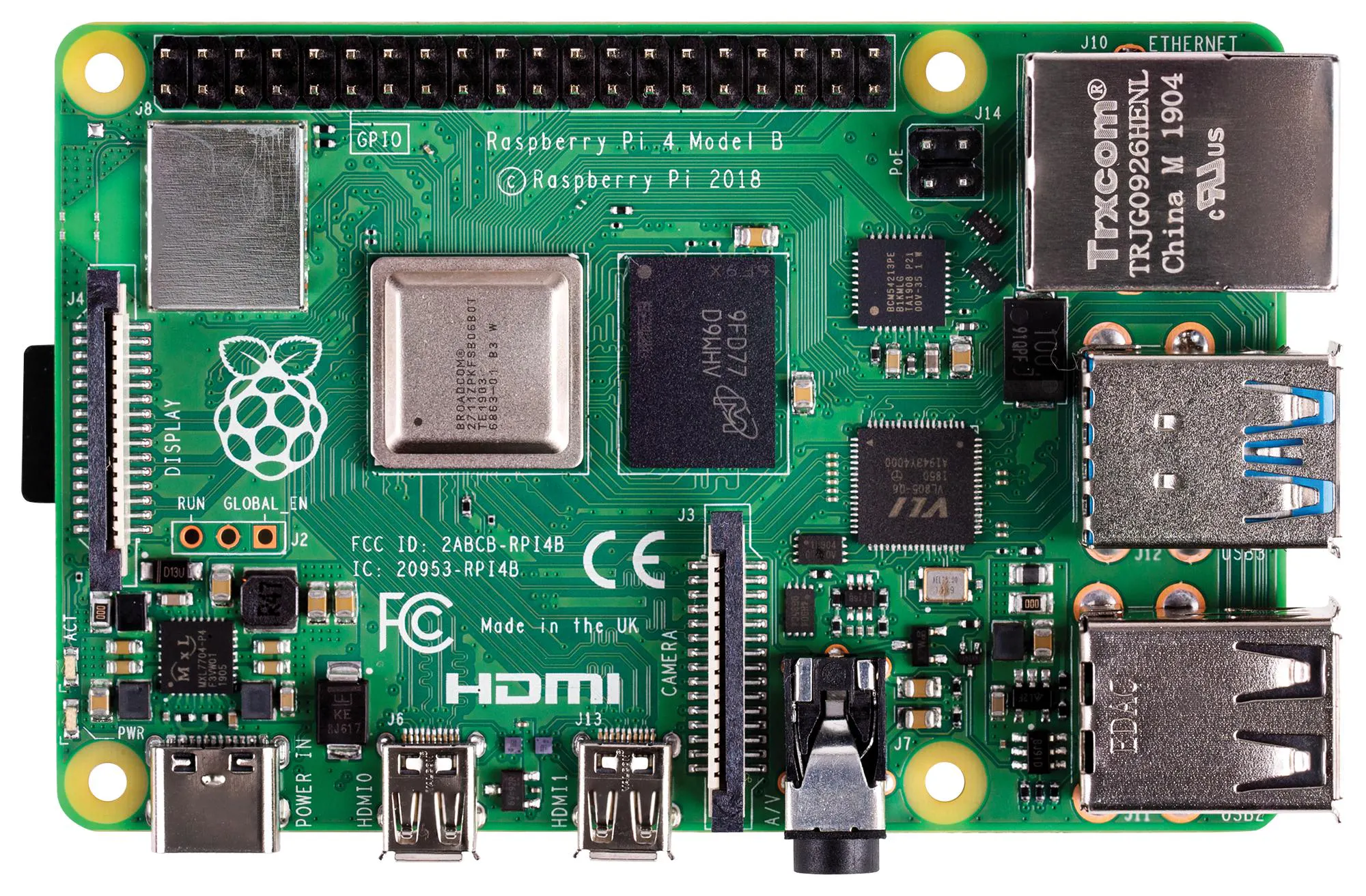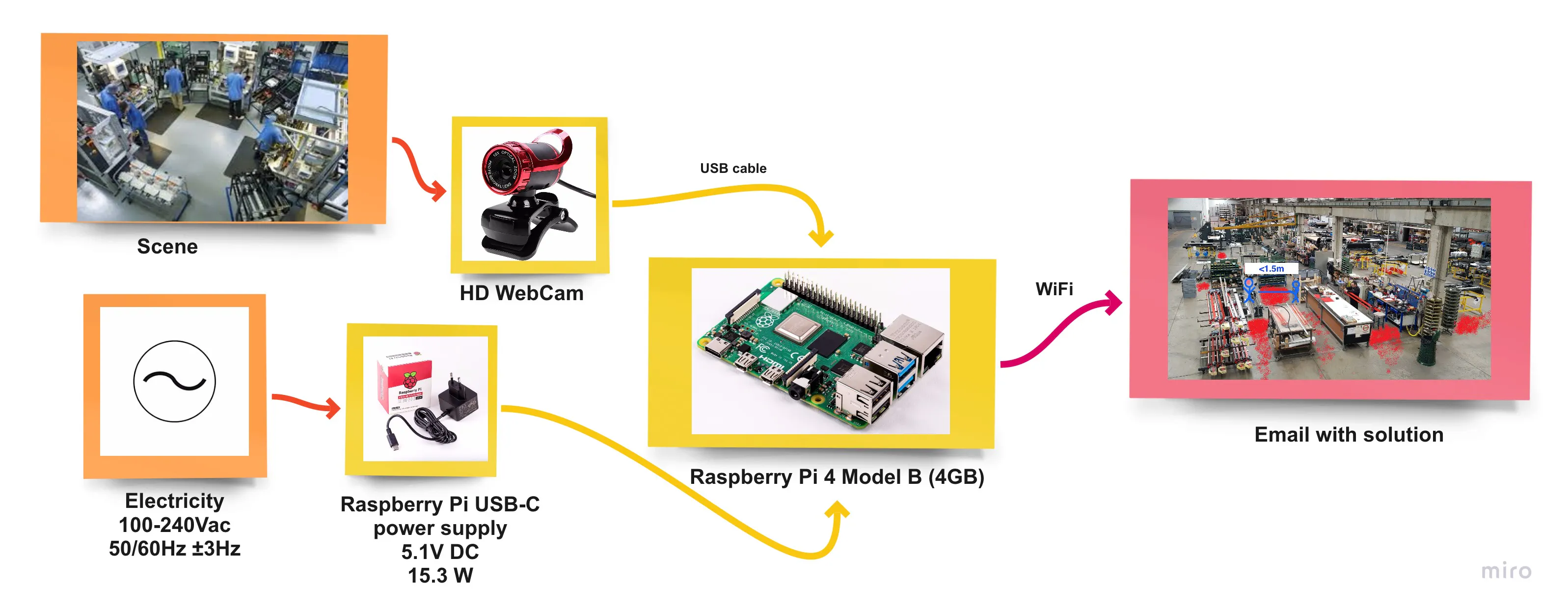# Safety Measures: Social Distancing

A contribution to maintaining a safe distance and the health of people in the time of COVID-19 crisis.

IntermediateFull instructions provided5 hours3,571

## Things used in this project

### Hardware componentsRaspberry Pi 4 Model B
×1
 Webcam YoLuke EL-PN-47320338
×1
 Raspberry Pi 15.3W USB-C Power Supply
×1

### Software apps and online services

 PyCharm

## Schematics

### Plan## Code

### Python Code

Python
```import cv2
import numpy as np
import time
import random
import math
from random import randrange

import smtplib
from email.mime.text import MIMEText
from email.mime.multipart import MIMEMultipart
from email.mime.base import MIMEBase
from email import encoders
import os.path

r"path-to/yolov3.cfg")
classes = []

#Importing classes, in this case only "person"
with open(r"path-to/coco.names", "r") as f:
classes = ["person"] #[line.strip() for line in f.readlines()]
layer_names = net.getLayerNames()
output_layers = [layer_names[i - 1] for i in net.getUnconnectedOutLayers()]
colors = np.random.uniform(0, 255, size=(len(classes), 3))

cap = cv2.VideoCapture(r"path-to/video.mp4")

length1 = int(cap.get(cv2.CAP_PROP_FRAME_COUNT))

font = cv2.FONT_HERSHEY_PLAIN
starting_time = time.time()
frame_id = 0
list_1 = []
list_2 = []
list_3 = []
list_4 = []

while(frame_id < length1):
print(frame_id)
frame_id += 3

height, width, channels = frame.shape

# Detecting objects
blob = cv2.dnn.blobFromImage(frame, 0.00392, (320, 320), (0, 0, 0), True, crop=False)

net.setInput(blob)
outs = net.forward(output_layers)

# Showing informations on the screen
class_ids = []
confidences = []
boxes = []
for out in outs:
for detection in out:
scores = detection[5:]
class_id = np.argmax(scores)
confidence = scores[class_id]
if confidence > 0.5:
# Object detected
center_x = int(detection * width)
center_y = int(detection * height)
w = int(detection * width)
h = int(detection * height)

# Rectangle coordinates
#x = int(center_x - w / 2) #for rectangle
#y = int(center_y - h / 2) #for rectangle
x = int(center_x)
y = int(center_y)
#cv2.circle(frame, (x, y), 1, (0, 255, 0), 3) #for circle
list_1.append([x, y])

boxes.append([x, y, w, h])
confidences.append(float(confidence))
class_ids.append(class_id)
list_2.append([x, y])

#Calculating the distance between people
for k3 in range(len(list_2)):
for k4 in range(len(list_2)):
if k3 != k4:
dx2_1 = (list_2[k3] - list_2[k4])**2
dy2_1 = (list_2[k3] - list_2[k4])**2
distance2 = math.sqrt(dx2_1 + dy2_1)
if distance2 < 50 and distance2 > 10:
dx2_2 = (list_2[k3] - list_2[k4]) / 2
dy2_2 = (list_2[k3] - list_2[k4]) / 2
x_1 = round(list_2[k4] + dx2_2)
y_1 = round(list_2[k4] + dy2_2)
list_3.append([x_1, y_1])
list_4.append([x_1, y_1])

#Drawing red dots if distance is too small
for k5 in range(len(list_4)):
cv2.circle(frame, (list_4[k5], list_4[k5]), 8, (0, 0, 255), 15)

indexes = cv2.dnn.NMSBoxes(boxes, confidences, 0.4, 0.3)

list_2.clear()
list_4.clear()

#Drawing paths of people
for i in range(len(boxes)):
if i in indexes:
x, y, w, h = boxes[i]
confidence = confidences[i]
g = random.randint(0, 255)
b = random.randint(0, 255)
r = random.randint(0, 255)
#color = colors[class_ids[i]]
#cv2.rectangle(frame, (x, y), (x + w, y + h), color, 2)
#cv2.rectangle(frame, (x, y), (x + w, y + 30), color, -1)
cv2.circle(frame, (x, y), 1, (0, 255, 0), 3)
#cv2.putText(frame, label + " " + str(round(confidence, 2)), (x, y + 30), font, 1, (255, 255, 255), 3)

elapsed_time = time.time() - starting_time
fps = frame_id / elapsed_time
cv2.putText(frame, "FPS: " + str(round(fps, 2)), (10, 50), font, 1, (0, 0, 0), 3)

cv2.imshow("Image", frame)
key = cv2.waitKey(1)
if key == 27:
break

#Drawing all paths in video
for k1 in range(len(list_1)):
for k2 in range(len(list_1)):
dx2 = (list_1[k1] - list_1[k2])**2
dy2 = (list_1[k1] - list_1[k2])**2
distance1 = math.sqrt(dx2 + dy2)
if distance1 < 15:
cv2.line(frame1, (list_1[k1], list_1[k1]), (list_1[k2], list_1[k2]), (0, 255, 0), 2)

cv2.imshow("Image", frame1)
#Saving image
cv2.imwrite(r"path-to\frame_name.jpg", frame1)

#Drawing all closer encounters in video
for k6 in range(len(list_3)):
cv2.circle(frame2, (list_3[k6], list_3[k6]), 8, (0, 0, 255), 15)

#Saving image
cv2.imwrite(r"path-to\frame_name2.jpg", frame2)

key = cv2.waitKey(10000)
cap.release()
cv2.destroyAllWindows()

#Writing email
email = 'sender mail'
send_to_email = 'receiving mail'
subject = 'This is the subject'
message = 'message'
file_location = r'path-to\frame1.jpg'
file_location = r'path-to\frame2.jpg'

msg = MIMEMultipart()
msg['From'] = email
msg['To'] = send_to_email
msg['Subject'] = subject

msg.attach(MIMEText(message, 'plain'))

# Setup the attachment
filename = os.path.basename(file_location)
attachment = open(file_location, "rb")
part = MIMEBase('application', 'octet-stream')
encoders.encode_base64(part)

# Setup the attachment 1
filename1 = os.path.basename(file_location1)
attachment1 = open(file_location1, "rb")
part1 = MIMEBase('application', 'octet-stream')
encoders.encode_base64(part1)

# Attach the attachment to the MIMEMultipart object
msg.attach(part)

server = smtplib.SMTP('smtp-mail.outlook.com', 587)
server.starttls()
text = msg.as_string()
server.sendmail(email, send_to_email, text)
server.quit()

# Attach the attachment to the MIMEMultipart object 1
msg.attach(part1)

server = smtplib.SMTP('smtp-mail.outlook.com', 587)
server.starttls()
text = msg.as_string()
server.sendmail(email, send_to_email, text)
server.quit()
```

## Credits

### Gašper Kastelic

1 project • 1 follower

### Nemanja Mihajlovic

0 projects • 3 followers

### Anže Dovžan Perović

0 projects • 2 followers

### Luka Mali

9 projects • 14 followers
Maker Pro, prototyping enthusiast, head of MakerLab, a lecturer at the University of Ljubljana, founder.